# PLOTTING with MATLAB

## simple 2D plot

h=0.2;
x=0:h:10;
y=sin(x);
plot(x,y);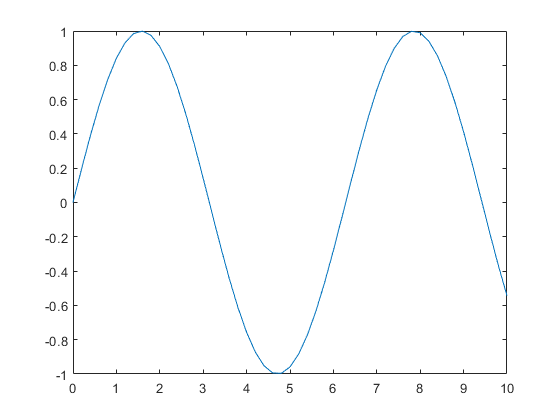To see the plot object characteristics you have to assign the plt to a variable:
pt=plot(x,y) % assign to a variable to see line propertiespt =
Line with properties: Color: [0 4.4700e-01 7.4100e-01] LineStyle: '-' LineWidth: 5.0000e-01 Marker: 'none' MarkerSize: 6 MarkerFaceColor: 'none' XData: [1×51 double] YData: [1×51 double] ZData: [1×0 double] Show all properties
plot(x,y,'LineWidth',1.5); % change line properties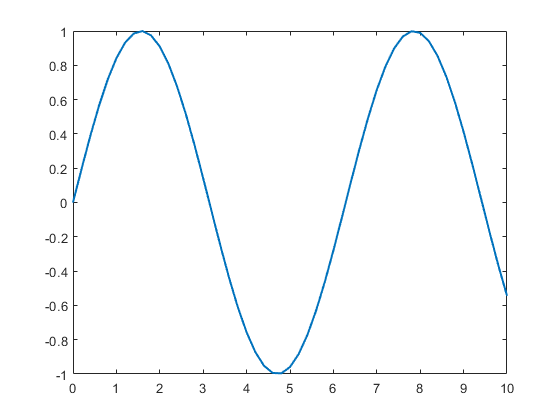plot(x,y,'LineStyle','--','LineWidth',1.5); % change line properties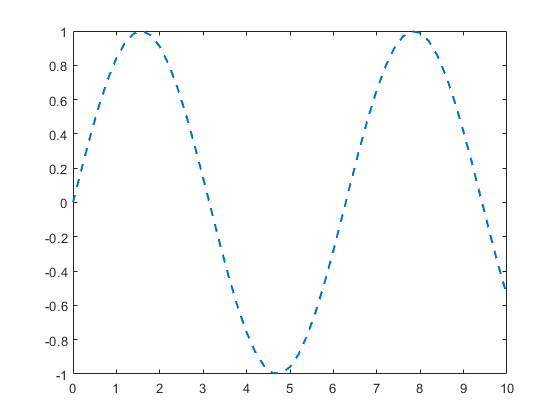## add more features: grid, title, etc.

h=0.2;
x=0:h:10;
y=sin(x);
plot(x,y);
axis([0,10,-1.1,1.1]) %give more room for the plot
grid %add grid
title('A nice plot') %add title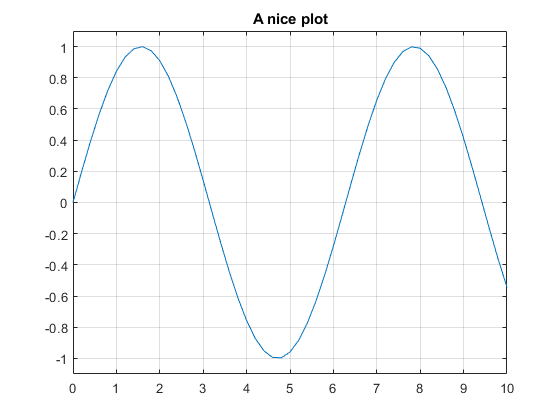## two plots in the same window

h=0.2;
x=0:h:10;
y=sin(x);
plot(x,y);
hold on;
z=cos(x);
plot(x,z);
axis([0,10,-1.1,1.1])
grid
title('Two plots')
legend('sin(x)','cos(x)') % to identify plots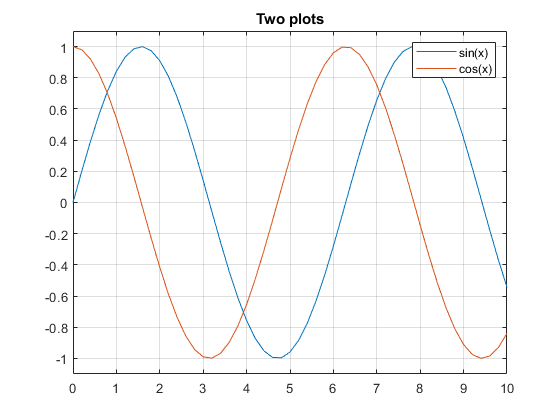## line style

h=0.2;
x=0:h:10;
y=sin(x);
z=cos(x);
plot (x, y,'-.k');
axis([0,10,-1.1,1.1])
title('Line styles')
hold on;
plot(x,z,'-sg') % lines and green squares (you can mixed)
grid
hold off;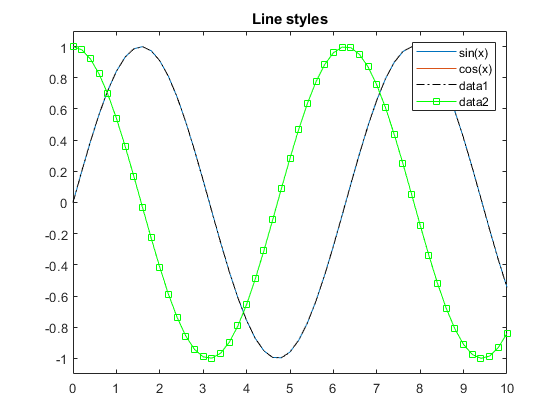In these tables you can find different line and marker options: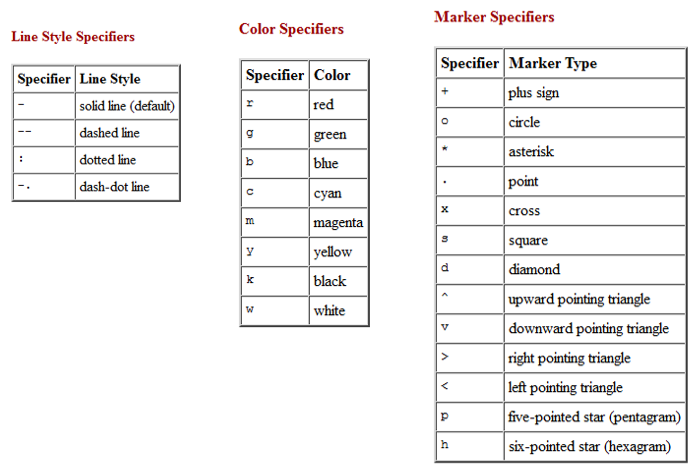## NaN terms are skipped when using plot

h=0.2;
x=-1:h:10;
y=sin(x)./x;
plot(x,y);
axis([-1,10,-1.1,1.1])
grid
title(' NaN skipped')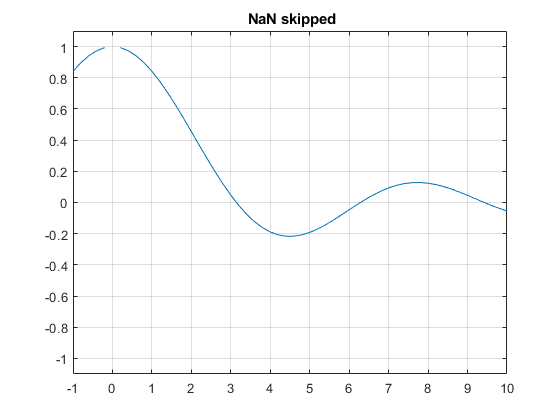## Plot a general function:

Let's plot the following functionYou have to define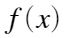like an inline function and then evaluate in a set of points
f=@(x) 10*(1-exp(-x/3).*sin(10*x));
h=0.1; % use only few points
xx=0:h:10;
yy=f(xx);
plot(xx,yy);
grid
title('Few points')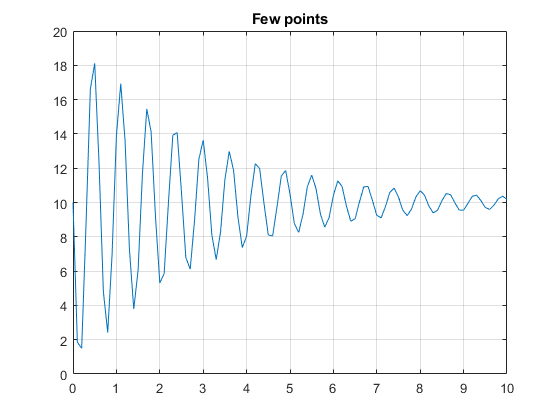h=0.02; % use more points
xx=0:h:10;
yy=f(xx);
plot(xx,yy);
%axis([0,10,-1.1,1.1])
grid
title('Enough points')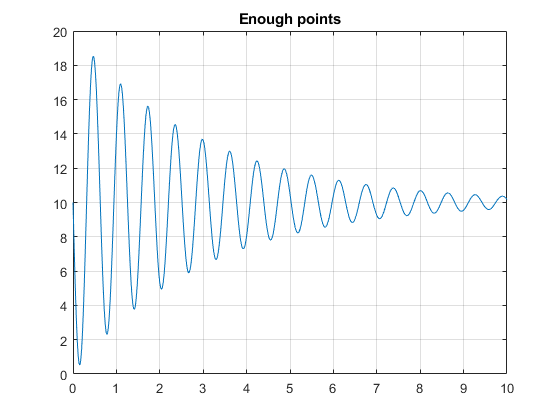## Subplots: many plots in the same window

subplot(2,2,1)
plot(x,y)
subplot(2,2,2) %empty plot (optional, you can comment it)
subplot(2,2,3) %empty plot (optional, you can comment it)
subplot(2,2,4)
plot(xx,yy)
hold off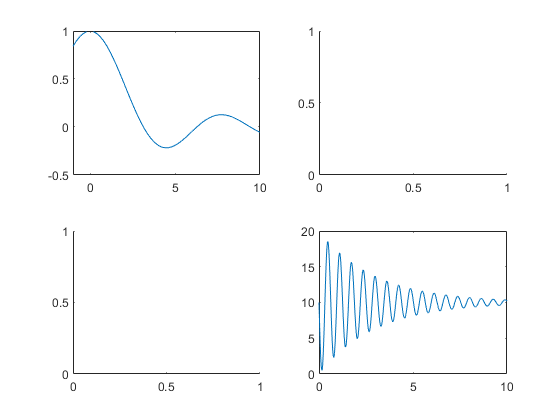## Get points with the mouse

Selecting 4 points with the mouse, you can obtain something like the image below.
% does not work, inside a livescript, for Matlab 2019 and previous versions
[x, y]=ginput(4); %click 4 times on the window
plot(x,y,'sm');
hold on;
fill(x,y,'r');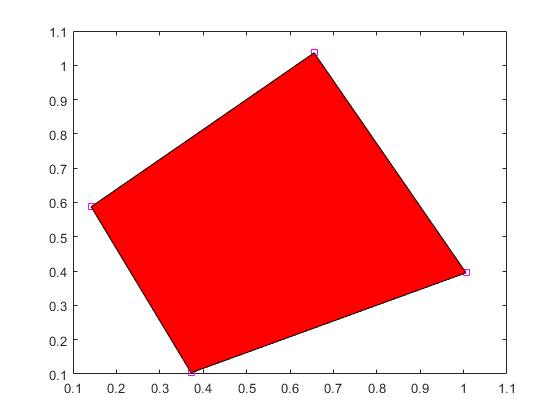## Other 2D plots

figure()
% Bar plot of a bell shaped curve
x = -2.9:0.2:2.9;
bar(x,exp(-x.^2));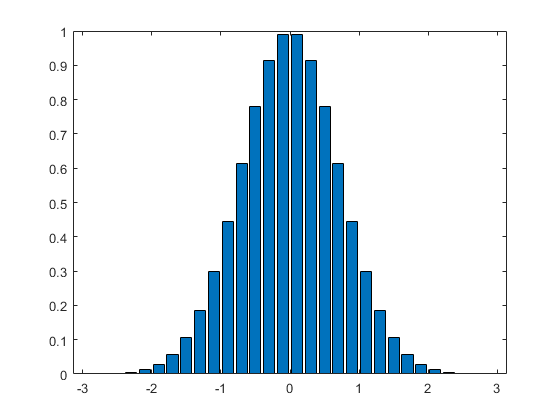% Stairstep plot of a sine wave
x=0:0.25:10;
stairs(x,sin(x));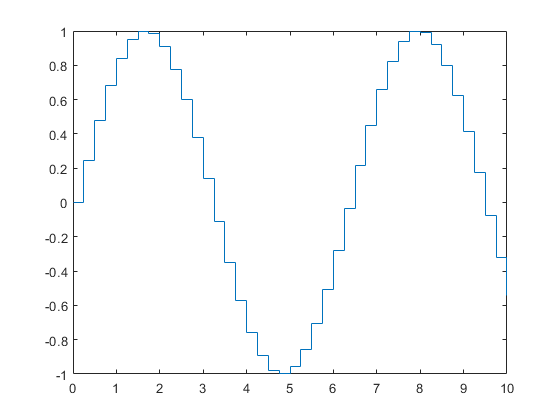% Errorbar plot
x=-2:0.1:2;
y=erf(x); %Matlab error function
e = rand(size(x))/10;
errorbar(x,y,e);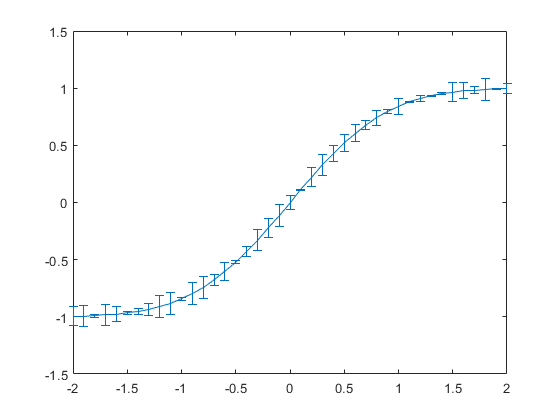% Polar plot
t=0:.01:2*pi;
polarplot(t,abs(sin(2*t).*cos(2*t)));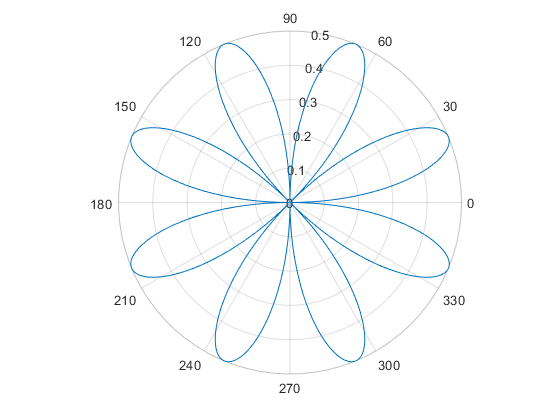% Stem plot
X = linspace(0,2*pi,50)';
Y = [cos(X), 0.5*sin(X)];
stem(Y)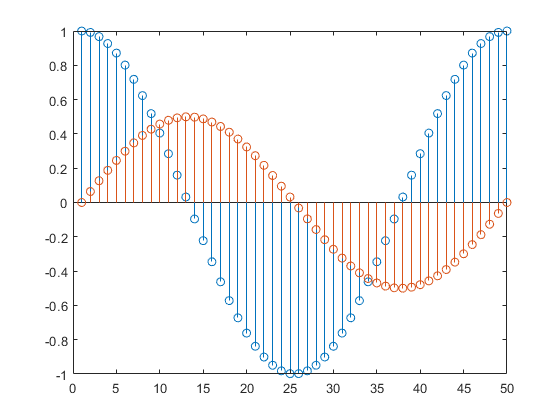## Animated Plots 2D: sinusoide

Sinusoid Animation: sin(x+P) where P is a phase value
%Assign values to the graphic window
figNumber=figure('Name','Sinusoid Animation', 'NumberTitle','off', 'Visible','on');
endP=2*pi; %max variation angle for P
incP=0.09; %increment de la Fase
x=-2*pi:0.2:2*pi; %rang of x values
for P=-endP:incP:endP
y=sin(x+P);
plot(x,y,'r')
hold on
axis([-2*pi 2*pi -1.2 1.2])
grid
plot([-2*pi 2*pi],[0 0],'Color','k','LineWidth',2)
plot([0 0],[-1.2 1.2],'Color','k','LineWidth',2)
text(1.15,1.1,['Phase = ' num2str(P,'%.4f')])
drawnow
%pause(0.01)
hold off;
end
hold off;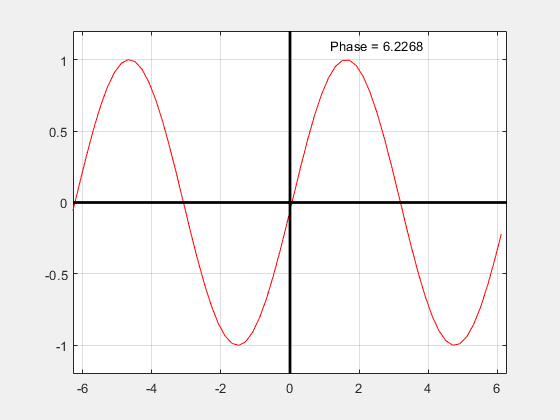## Animated Plots 3D: Helix Animation

figure()
fi=0:pi/20:6*pi;
for k=1:4:size(fi,2)
plot3(cos(fi),sin(fi),fi,'b')
hold on;
plot3(cos(fi(k)), sin(fi(k)), fi(k),'marker','o',...
'markersize',8,'markerfacecolor', 'green');
hold off;
pause(0.1)
end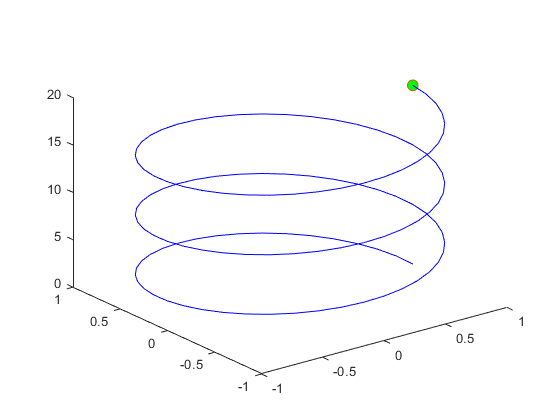## Create a Video from an animation:

recordVideo=0; %=0 to see it on the screen, =1 to record the video
if (recordVideo==1)
myVideo= VideoWriter('helix.avi'); %name for the video file
open(myVideo); %open our video file
end
fi=0:pi/20:6*pi;
for k=1:size(fi,2)
plot3(cos(fi),sin(fi),fi,'b')
hold on;
plot3(cos(fi(k)), sin(fi(k)), fi(k),'marker','o',...
'markersize',8,'markerfacecolor', 'green');
hold off;
drawnow
pause(0.01)
if (recordVideo==1)
frame = getframe; %get the present plot
writeVideo(myVideo,frame); %save it to the video
end
endif (recordVideo==1), close(myVideo); end

## Mesgrid

x=-2:2;
y=-4:4;
[X,Y]=meshgrid(x,y)
X = 9×5
-2 -1 0 1 2 -2 -1 0 1 2 -2 -1 0 1 2 -2 -1 0 1 2 -2 -1 0 1 2 -2 -1 0 1 2 -2 -1 0 1 2 -2 -1 0 1 2 -2 -1 0 1 2
Y = 9×5
-4 -4 -4 -4 -4 -3 -3 -3 -3 -3 -2 -2 -2 -2 -2 -1 -1 -1 -1 -1 0 0 0 0 0 1 1 1 1 1 2 2 2 2 2 3 3 3 3 3 4 4 4 4 4
z=X.^2+Y.^2;
figure()
surf(X,Y,z)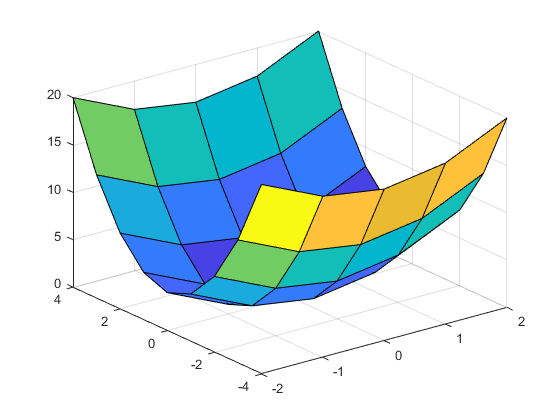figure()
plot(X,Y,'o')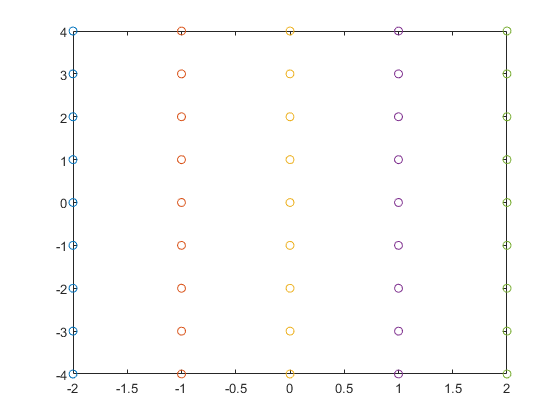## 3D surfaces

x=-8:0.6:8;
y=x;
[X,Y]=meshgrid(x,y);
R=sqrt(X.^2 +Y.^2);
W= sin(R)./R;
mesh(X,Y,W);